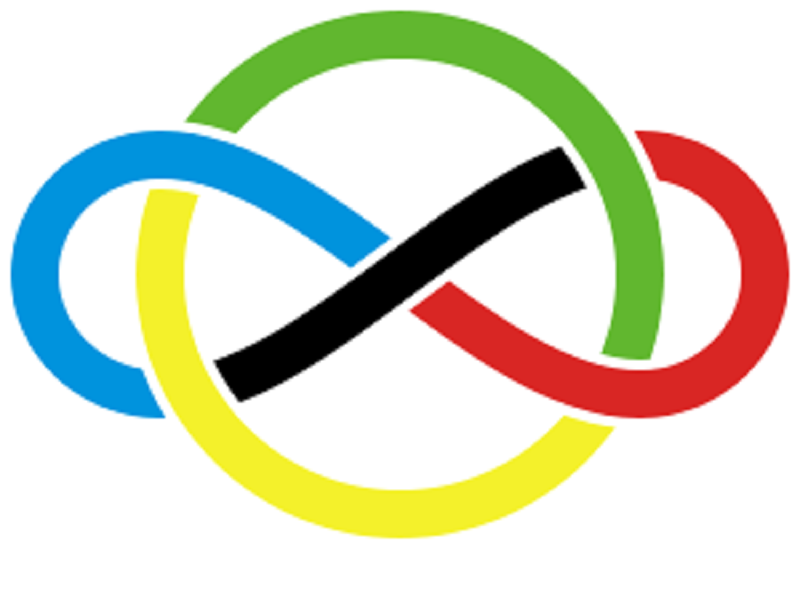### Unlimited Printable Worksheets, Online Practice and Online Tests For IMOThe International Mathematical Olympiad (IMO) is the World Championship Mathematics Competition for High School students and is held annually in a different countries.

Though exam is conducted for high-school students, the tests in this category help students prepare for this exam from early grades.

### 1

Numbers, Addition, Subtraction, Measurement, Patterns, Odd One Out, Shapes, Time

### 2

Numbers, Computational Operations, Measurement, Time, Shapes and Solids, Pictographs, Patterns, Odd One Out, Analogy

### 3

Numbers, Computation Operations, Fractions, Money, Measurement, Geometry, Data Handling, Time, Patterns, Analogy, Mirror Images, Embedded Figures

### 4

Numbers, Computational Operations, Fractions and Decimals, Measurement, Time, Money, Geometry, Perimeter and Area, Symmetry and Patterns, Data Handling, Mirror Images, Embedded Figures

### 5

Numbers, Computational Operations, Fractions and Decimals, Measurement, Time, Money, Geometry, Perimeter and Area, Symmetry and Patterns, Data Handling, Mirror Images, Embedded Figures

### 6

Numbers, Integers, Fractions and Decimals, Algebra, Mensuration, Geometry, Ratio and Proportion, Symmetry, Data Handling

### 7

Integers, Fractions and Decimals, Exponents and Powers, Algebra, Percenatge, Simple Interest, Triangle, Geometry, Perimeter and Area, Data Handling

### 8

Rational Numbers, Squares and Square Roots, Cubes and Cube Roots, Exponents and Powers, Percentage, Simple and Compound Interest, Algebra, Mensuration, Geometry, Direct and Inverse Variations, Graphs